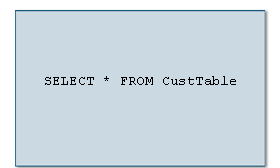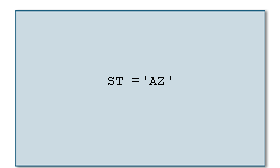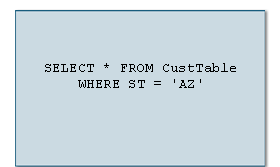Basic Queries  «Prev  Next»
 Lesson 3 SQL WHERE clause Objective Use WHERE to select specific rows of a table.

## SQL WHERE Clause

Often times you will want queries to return only a subset of the entire database. For example, when searching for a list of books by a specific author. The SELECT statement's WHERE clause allows you to select only data from rows that satisfy a certain condition. A clause further defines a statement. For example the following statement returns all rows:

```SELECT * FROM BookTable
```
Adding the WHERE clause such as WHERE Author='Hemingway', further defines the statement to return only rows in which the author equals Hemingway. The following SQL query returns books by Hemingway.
```SELECT * FROM BookTable WHERE Author = 'Hemingway'
```

The WHERE clause uses comparison and logical operators to select rows meeting the specified condition. The following SlideShow describes how the WHERE clause is used:Using Where Clause
SQL has several operators that allow the SELECT statement to be very selective about the data returned from a query. The following tables list the comparison and logical operators used with SQL:

1
 Comparison Operators Symbol Function = Equal <> Not equal > Greater than >= Greater than or equal < Less than <= Less than or equal

 Logical Operators Operator Function AND Returns TRUE if all conditions are true OR Returns TRUE if one or more conditions are true NOT Returns TRUE if the condition is false

The following statement uses the logical operator AND to select rows where state equals Arizona and last name equals Henry:

```SELECT * FROM CustTable WHERE ST = 'AZ' AND
LName = 'Henry'
```
In the next lesson, the ORDER BY clause to sort rows in a table will be discussed.